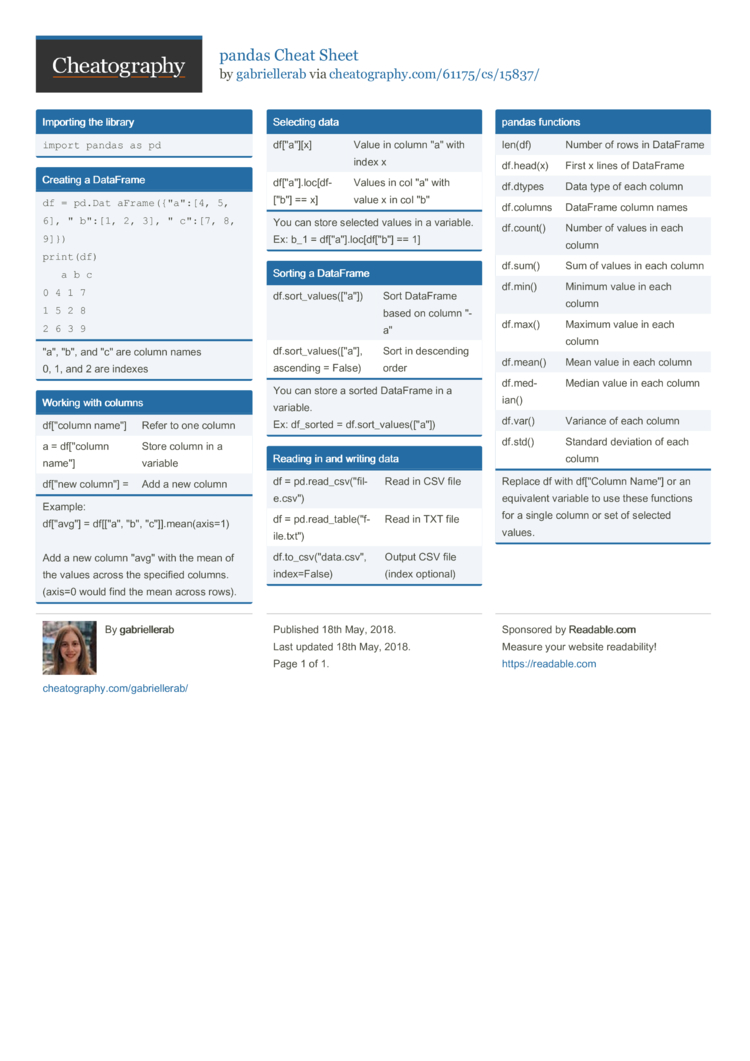pandas Cheat Sheet by gabriellerab

Importing the library

 import pandas as pd

Creating a DataFrame

 df = pd.Dat­aFrame({"a":[4, 5, 6], "­b":[1, 2, 3], "­c":[7, 8, 9]}) print(df)   a b c 0 4 1 7 1 5 2 8 2 6 3 9
"­a", "­b", and "­c" are column names
0, 1, and 2 are indexes

Working with columns

 df["­column name"] Refer to one column a = df["­column name"] Store column in a variable df["new column­"] = Add a new column
Example:
df["­avg­"] = df[["a", "­b", "­c"]].me­an(­axis=1)

Add a new column "­avg­" with the mean of the values across the specified columns.
(axis=0 would find the mean across rows).

Selecting data

 df["­a"][x] Value in column "­a" with index x df["­a"].l­oc­[df­["b"] == x] Values in col "­a" with value x in col "­b"
You can store selected values in a variable. Ex: b_1 = df["­a"].l­oc­[df­["b"] == 1]

Sorting a DataFrame

 df.sor­t_v­alu­es(­["a"]) Sort DataFrame based on column "­a" df.sor­t_v­alu­es(­["a"], ascending = False) Sort in descending order
You can store a sorted DataFrame in a variable.
Ex: df_sorted = df.sor­t_v­alu­es(­["a"])

Reading in and writing data

 df = pd.rea­d_c­sv(­"­fil­e.c­sv") Read in CSV file df = pd.rea­d_t­abl­e("f­ile.tx­t") Read in TXT file df.to_­csv­("da­ta.c­sv­", index=­False) Output CSV file (index optional)

pandas functions

 len(df) Number of rows in DataFrame df.head(x) First x lines of DataFrame df.dtypes Data type of each column df.columns DataFrame column names df.count() Number of values in each column df.sum() Sum of values in each column df.min() Minimum value in each column df.max() Maximum value in each column df.mean() Mean value in each column df.med­ian() Median value in each column df.var() Variance of each column df.std() Standard deviation of each column
Replace df with df["­Column Name"] or an equivalent variable to use these functions for a single column or set of selected values.1 Page
//media.cheatography.com/storage/thumb/gabriellerab_pandas.750.jpg

PDF (recommended)